# RD Sharma Solutions for Class 11 Chapter 23 - The Straight Lines Exercise 23.11

This exercise discusses the condition of concurrency of three lines with examples and illustrations for students to grasp the concepts clearly. Students can obtain a good score in their examination by using the solution module developed by our subject experts at BYJU’S. The solutions here are developed using shortcut techniques, which makes learning fun and easy. For further reference, students can make use of RD Sharma Class 11 Maths Solutions, which helps students effortlessly prepare for their exams. Students can refer and easily download the pdf for free, from the links given below.

## Download the pdf of RD Sharma Solutions for Class 11 Maths Exercise 23.11 Chapter 23 – The Straight Lines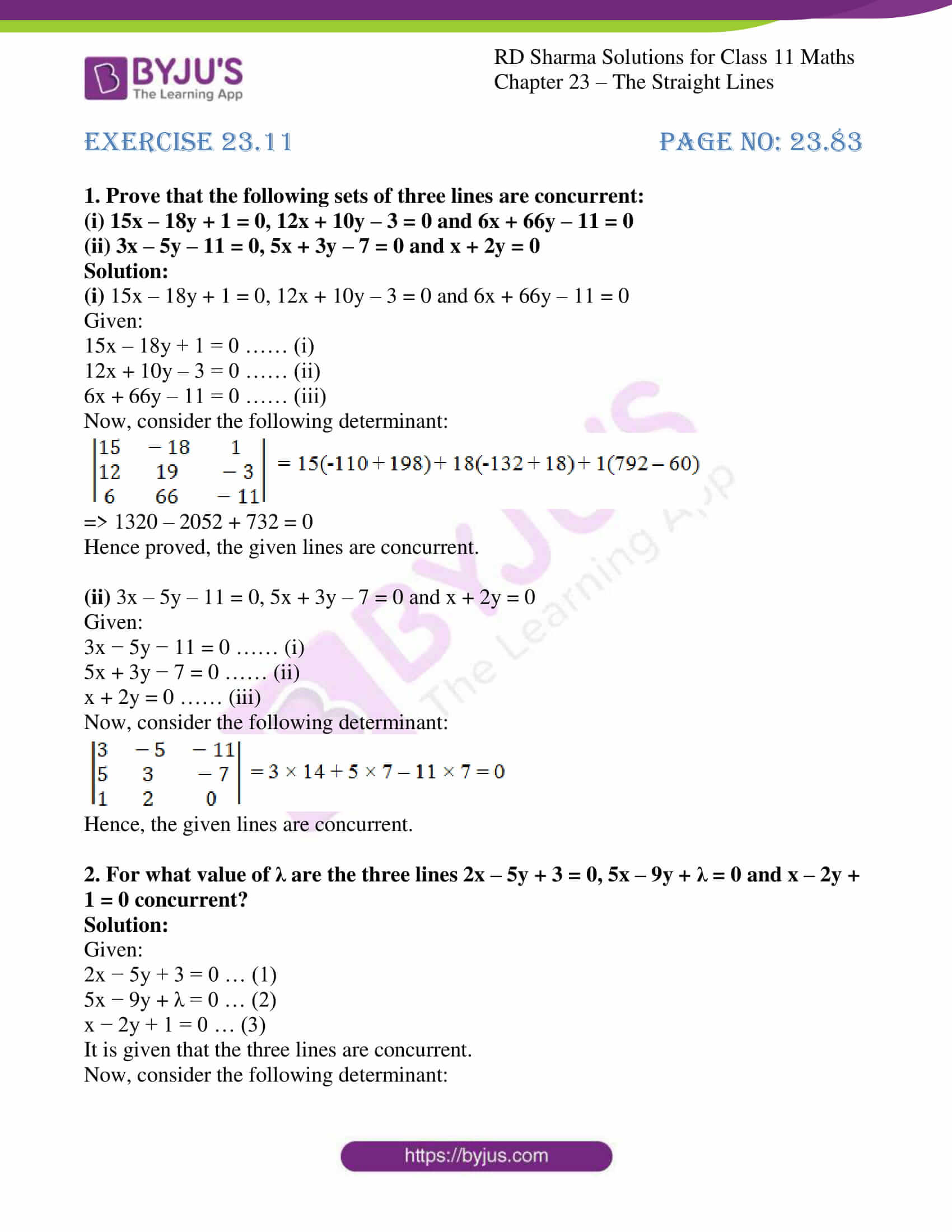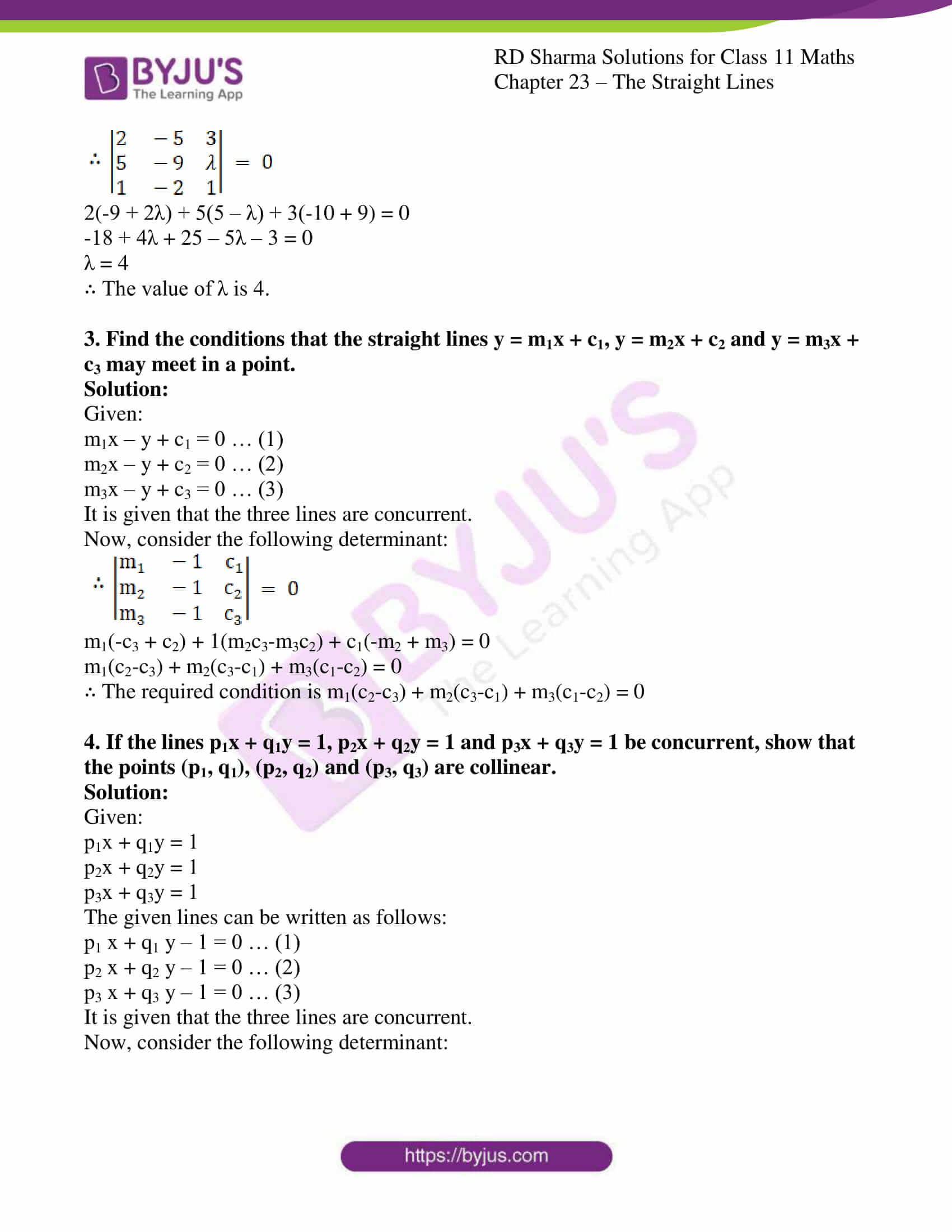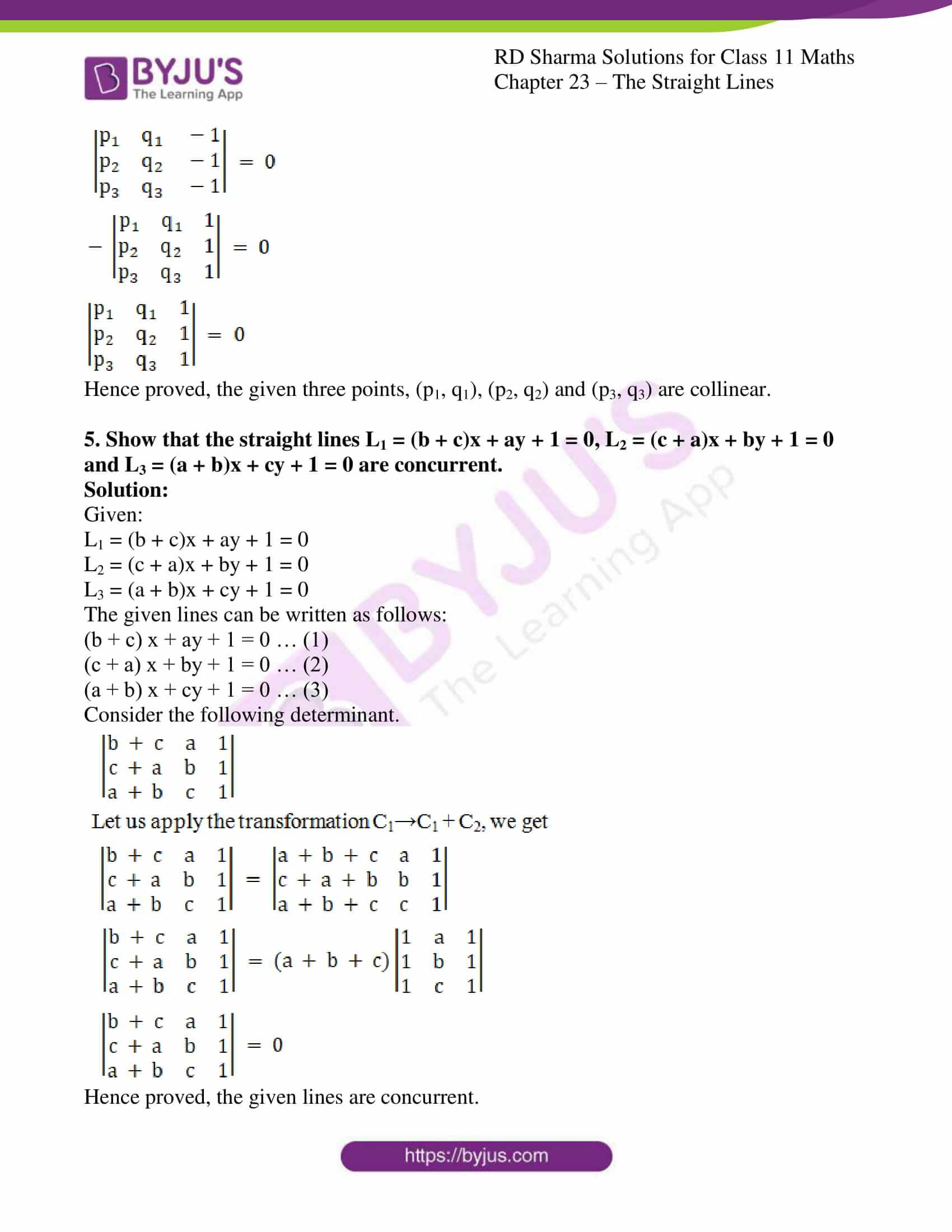### Also, access other exercises of RD Sharma Solutions for Class 11 Maths Chapter 23 – The Straight Lines

Exercise 23.1 Solutions

Exercise 23.2 Solutions

Exercise 23.3 Solutions

Exercise 23.4 Solutions

Exercise 23.5 Solutions

Exercise 23.6 Solutions

Exercise 23.7 Solutions

Exercise 23.8 Solutions

Exercise 23.9 Solutions

Exercise 23.10 Solutions

Exercise 23.12 Solutions

Exercise 23.13 Solutions

Exercise 23.14 Solutions

Exercise 23.15 Solutions

Exercise 23.16 Solutions

Exercise 23.17 Solutions

Exercise 23.18 Solutions

Exercise 23.19 Solutions

### Access answers to RD Sharma Solutions for Class 11 Maths Exercise 23.11 Chapter 23 – The Straight Lines

#### EXERCISE 23.11 PAGE NO: 23.83

1. Prove that the following sets of three lines are concurrent:
(i) 15x – 18y + 1 = 0, 12x + 10y – 3 = 0 and 6x + 66y – 11 = 0

(ii) 3x – 5y – 11 = 0, 5x + 3y – 7 = 0 and x + 2y = 0

Solution:

(i) 15x – 18y + 1 = 0, 12x + 10y – 3 = 0 and 6x + 66y – 11 = 0

Given:

15x – 18y + 1 = 0 …… (i)

12x + 10y – 3 = 0 …… (ii)

6x + 66y – 11 = 0 …… (iii)

Now, consider the following determinant: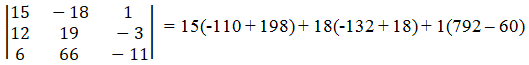=> 1320 – 2052 + 732 = 0

Hence proved, the given lines are concurrent.

(ii) 3x – 5y – 11 = 0, 5x + 3y – 7 = 0 and x + 2y = 0

Given:

3x − 5y − 11 = 0 …… (i)

5x + 3y − 7 = 0 …… (ii)

x + 2y = 0 …… (iii)

Now, consider the following determinant: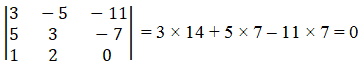Hence, the given lines are concurrent.

2. For what value of λ are the three lines 2x – 5y + 3 = 0, 5x – 9y + λ = 0 and x – 2y + 1 = 0 concurrent?

Solution:

Given:

2x − 5y + 3 = 0 … (1)

5x − 9y + λ = 0 … (2)

x − 2y + 1 = 0 … (3)

It is given that the three lines are concurrent.

Now, consider the following determinant: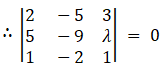2(-9 + 2λ) + 5(5 – λ) + 3(-10 + 9) = 0

-18 + 4λ + 25 – 5λ – 3 = 0

λ = 4

∴ The value of λ is 4.

3. Find the conditions that the straight lines y = m1x + c1, y = m2x + c2 and y = m3x + c3 may meet in a point.

Solution:

Given:

m1x – y + c1 = 0 … (1)

m2x – y + c2 = 0 … (2)

m3x – y + c3 = 0 … (3)

It is given that the three lines are concurrent.

Now, consider the following determinant: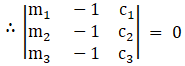m1(-c3 + c2) + 1(m2c3-m3c2) + c1(-m2 + m3) = 0

m1(c2-c3) + m2(c3-c1) + m3(c1-c2) = 0

∴ The required condition is m1(c2-c3) + m2(c3-c1) + m3(c1-c2) = 0

4. If the lines p1x + q1y = 1, p2x + q2y = 1 and p3x + q3y = 1 be concurrent, show that the points (p1, q1), (p2, q2) and (p3, q3) are collinear.

Solution:

Given:

p1x + q1y = 1

p2x + q2y = 1

p3x + q3y = 1

The given lines can be written as follows:

p1 x + q1 y – 1 = 0 … (1)

p2 x + q2 y – 1 = 0 … (2)

p3 x + q3 y – 1 = 0 … (3)

It is given that the three lines are concurrent.

Now, consider the following determinant: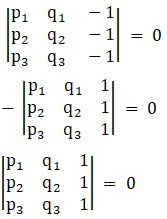Hence proved, the given three points, (p1, q1), (p2, q2) and (p3, q3) are collinear.

5. Show that the straight lines L1 = (b + c)x + ay + 1 = 0, L2 = (c + a)x + by + 1 = 0 and L3 = (a + b)x + cy + 1 = 0 are concurrent.

Solution:

Given:

L1 = (b + c)x + ay + 1 = 0

L2 = (c + a)x + by + 1 = 0

L3 = (a + b)x + cy + 1 = 0

The given lines can be written as follows:

(b + c) x + ay + 1 = 0 … (1)

(c + a) x + by + 1 = 0 … (2)

(a + b) x + cy + 1 = 0 … (3)

Consider the following determinant.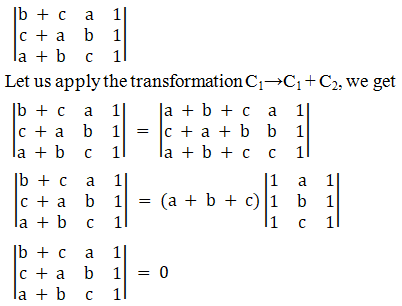Hence proved, the given lines are concurrent.